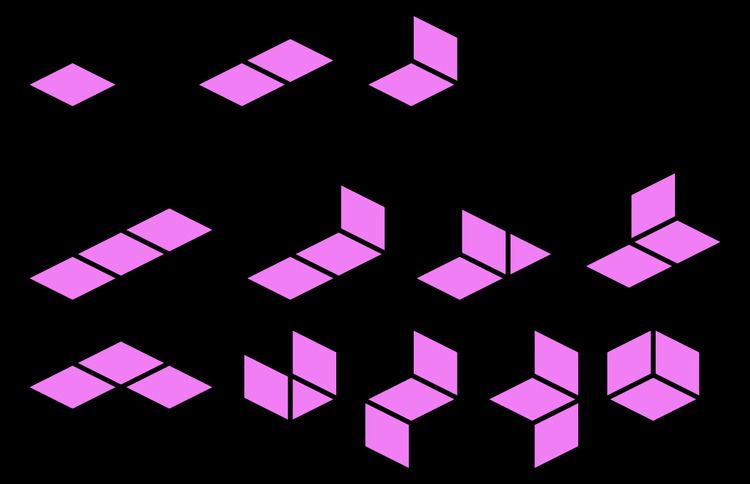# Polyominoid

Updated onIn geometry, a polyominoid (or minoid for short) is a set of equal squares in 3D space, joined edge to edge at 90- or 180-degree angles. The polyominoids include the polyominoes, which are just the planar polyominoids. The surface of a cube is an example of a hexominoid, or 6-cell polyominoid, and many other polycubes have polyominoids as their boundaries. Polyominoids appear to have been first proposed by Richard A. Epstein.

## Classification

90-degree connections are called hard; 180-degree connections are called soft. This is because, in manufacturing a model of the polyominoid, a hard connection would be easier to realize than a soft one. Polyominoids may be classified as hard if every junction includes a 90° connection, soft if every connection is 180°, and mixed otherwise, except that the unique monominoid has no connections of either kind, which makes it both hard and soft by default. The soft polyominoids are just the polyominoes.

As with other polyforms, two polyominoids that are mirror images may be distinguished. One-sided polyominoids distinguish mirror images; free polyominoids do not.

## Enumeration

The table below enumerates free and one-sided polyominoids of up to 6 cells.

## Generalization to higher dimensions

In general one can define an n,k-polyominoid as a polyform made by joining k-dimensional hypercubes at 90° or 180° angles in n-dimensional space, where 1≤kn.

• Polysticks are 2,1-polyominoids.
• Polyominoes are 2,2-polyominoids.
• The polyforms described above are 3,2-polyominoids.
• Polycubes are 3,3-polyominoids.
• ## References

Similar Topics
The Flying Serpent